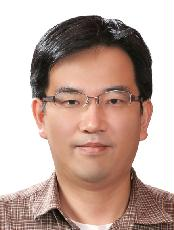###정보이름최영우 학력박사 전공수학전공(과) 연구실 : 팔달관718호 연구실번호 : 2570 이메일 : youngwoo@ajou.ac.kr 연구관심분야 : 조화해석학 홈페이지 : 졸업연도/학교/학위 1995.12 University of Wisconsin-Madison 박사 졸업연도/학교/학위 1991.02 서울대학교 학사 연구활동(주요논문) [논문] Totally interpolating multiwavelet systems with approximation order (Linear and Multilinear Algebra) - 2012.06 최영우, 정재원 [논문] The L^p-L^q mapping properties of convolution operators with the affine arclength measure on space curves (Journal of the Australian Mathematical Society) - 2003.10 최영우 [논문] Marcinkiewicz integrals with rough homogeneous kernels of degree zero in product domains (Journal of Mathematical Analysis and Applications) - 2001.08 최영우 [논문] Damping oscillatory integrals with polynomial phase and convolution operators with the affine arclength measure on polynomial curves in R^n (Journal of the London Mathematical Society) - 2000.02 최영우 [논문] Convolution operators with the affine arclength measure on plane curves (대한수학회지 (J. Korean Math. Soc.)) - 1999.01 최영우 국제학술논문지 [논문] Uniform estimates for damped Radon transform on the plane (ABSTRACT AND APPLIED ANALYSIS) - 2013.10 최영우 [논문] Totally interpolating multiwavelet systems with approximation order (Linear and Multilinear Algebra) - 2012.06 최영우, 정재원 [논문] APPROXIMATION AND BALANCING ORDERS FOR TOTALLY INTERPOLATING BIORTHOGONAL MULTIWAVELET SYSTEMS (BULLETIN OF THE KOREAN MATHEMATICAL SOCIETY) - 2011.11 최영우, 정재원 [논문] Convolution estimates related to space curves (JOURNAL OF INEQUALITIES AND APPLICATIONS) - 2011.10 최영우 [논문] The L^p-L^q mapping properties of convolution operators with the affine arclength measure on space curves (Journal of the Australian Mathematical Society) - 2003.10 최영우 [논문] Marcinkiewicz integrals with rough homogeneous kernels of degree zero in product domains (Journal of Mathematical Analysis and Applications) - 2001.08 최영우 [논문] Damping oscillatory integrals with polynomial phase and convolution operators with the affine arclength measure on polynomial curves in R^n (Journal of the London Mathematical Society) - 2000.02 최영우 국내학술논문지 [논문] Mapping properties of the Marcinkiewicz integrals on homogeneous groups (Journal of the Korean Mathematical Society) - 2002.01 최영우, 임경수 [논문] Convolution operators with the affine arclength measure on plane curves (대한수학회지 (J. Korean Math. Soc.)) - 1999.01 최영우